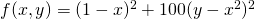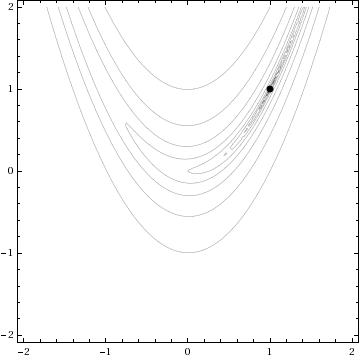## Minimising the Rosenbrock Function using Mathematica

April 9th, 2008 | Categories: general math, math software, mathematica, Wolfram Demonstrations | Tags:

The minimization of the Rosenbrock function is a classic test problem that is extensively used to test the performance of different numerical optimization algorithms. It was first introduced in 1960 by H.H Rosenbrock in his paper, “An Automatic Method for Finding the Greatest or Least Value of a Function, Computer J. 3, 175-184, 1960 (See here for Rosenbrock’s account of how he came to write that paper.)

The function definition is given below and, although it looks harmless enough, it turns out to be quite challenging to find it’s minimum point by numerical methods.Acording to Google Scholar, Rosenbrock’s paper has been cited no less than 596 times (as of April 9th 2008) and the function he introduced has been used as a test problem for many different numerical solvers including those in the Matlab Optimization toolbox, The NAG libraries, COMSOL and SciPy (A python module).

So why is this innocuous looking function so difficult to minimise? To answer that let’s first take a look at its contour plot using Mathematica.

ContourPlot[(1 – x)^2 + 100 (y – x^2)^2, {x, -2, 2}, {y, -2, 2},
ContourShading -> False, Contours -> Table[10^-i, {i, -2, 10, 0.5}]
, Epilog -> {Black, PointSize[Large], Point[{1, 1}]}]The global minimum of the Rosenbrock function lies at the point x=1, y=1 and is shown in the above diagram by a black spot. Note that the contours are not evenly spaced in this diagram – they are logarithmically spaced instead so the solution lies inside a very deep, narrow, banana shaped valley. The distinctive shape of this function’s contour lines is the reason for its alternative name – “Rosenbrock’s banana function”.

The valley causes a lot of problems for search algorithms such as the method of steepest descents which will quickly find the ‘entrance’ to the valley and then spend hundreds of iterations zigzagging from one side of it to the other – making very slow progress towards the minimum itself.

There is a Mathematica function called FindMinimum that can handle this kind of optimization problem and I wondered how its methods would handle the Rosenbrock function. In the back of my mind I thought that I might also be able to make a nice Wolfram Demonstration from the investigation. While looking at the documentation for FindMinimum I found an example that pretty much solved the problem for me.

pts = Reap[FindMinimum[(1 – x)^2 + 100 (-x^2 – y)^2, {{x, -1.2}, {y, 1}}, StepMonitor :> Sow[{x, y}]]][[2, 1]];
pts = Join[{{-1.2, 1}}, pts];

This finds the minimum point from a starting point of x=-1.2, y=1 and also stores all of the intermediate steps so you can see the path that Mathematica takes in looking for the solution. The only other piece of information needed was to find out how to specify the solution method. It turns out that this is obvious – just use the option

Method->”MethodNameHere”

replacing MethodNameHere with whatever method you want Mathematica to use. You can choose from ConjugateGradient, LevenbergMarquardt, Newton, QuasiNewton, PrincipalAxis and InteriorPoint.

All that remained was to wrap this up in a Manipulate statement, make it look pretty, add some controls and submit it to the demonstrations project. The result can be found here.

One of the things I like about the process of submitting demonstrations to the project is that they are refereed. Someone will take the time to look at your code and make suggestions and small modifications before it gets published. This reduces the possibility of mistakes being made in the final version and really helps with the learning process. Almost every time I have submitted something, I have learned a little more about Mathematica – and that’s really the main reason for me doing it (well its also fun to have your name “up in lights” if I am being honest).

At this point I would like to thank the members of the Wolfram Demonstrations team who have dealt with my submissions so far – not only have they been a pleasure to work with and extremely patient but they have also spared my blushes by pointing out some very stupid mistakes in my code. Of course any that remain are my fault and not theirs.